# LEG THICKNESS LIMITS BASED ON PIVOTING RANGES AND WELL PERIMETER EDGE

The thickness of the outermost telescoping leg segment (the rectangular sides of a cross section) determines the leg’s pivoting range limit. For a thicker leg the pivoting range limit is smaller, and for a thinner leg the pivoting range limit is larger. The tradeoffs for a thicker leg include the following features:

1. more strength against leg bending strain or damage
2. more space for the mechanical system to telescope the leg
3. more leg segments for telescoping the leg

For both Figures 21a and 21b the variable a and the module core assembly base component ratios are based on the calculations from the Optimal Ratio Calculation for Module Core Assembly Base Component article.Figure 21a is a diagram of a lengthwise cross section of the base of the module that forms the module core assembly base component as a black outline and a representation of the boundary of the leg that can enter the well as a blue line. The lengthwise well perimeter is indicated by the red vertical lines. The variable L represents half the lengthwise thickness of the leg, and the variable θL represents the lengthwise plane of rotation angle for the leg from a perpendicular position relative to the face of the cell cube.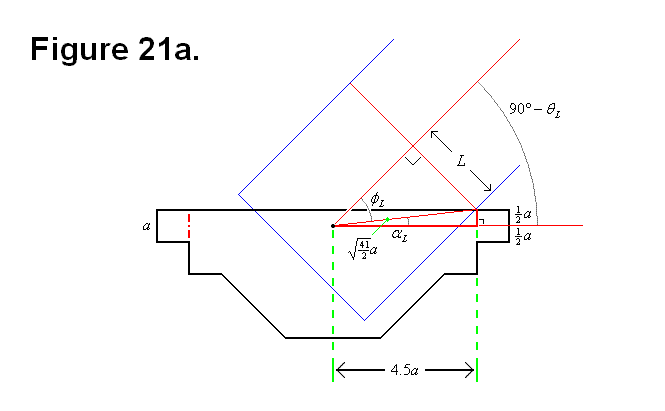The following equations and calculations are derived from the geometrical information provided by Figure 21a: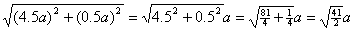(Equation #1)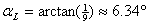(Equation #2)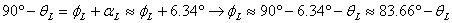(Formula #1)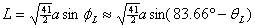(Formula #2)

Figure 21b is a diagram of a widthwise cross section of the base of the module that forms the module core assembly base component as a black outline and a representation of the boundary of the leg that can enter the well as a blue line. The widthwise well perimeter is indicated by the red vertical lines. The variable W represents half the widthwise thickness of the leg, and the variable θW represents the widthwise plane of rotation angle for the leg from a perpendicular position relative to the face of the cell cube.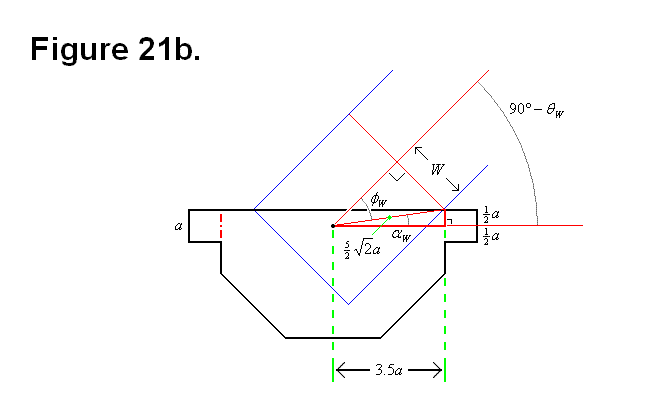The following equations and calculations are derived from the geometrical information provided by Figure 21b: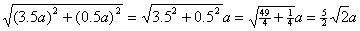(Equation #3)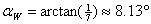(Equation #4)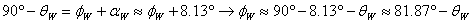(Formula #3)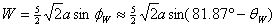(Formula #4)
Graph #1 Graph #2
Graph # 1 shows a plot of the angle versus the rectangular lenghwise thickness factor (to ratio variable a) of the leg. Green is for an angle of 45 degrees, and red is for an angle of 60 degrees. Beyond 60 degrees the decrease is almost linear.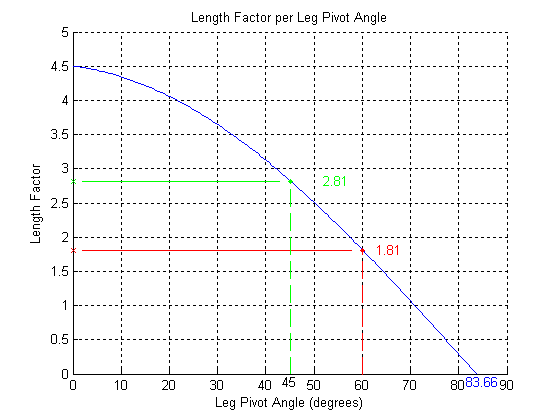Graph # 2 shows a plot of the angle versus the rectangular widthwise thickness factor (to ratio variable a) of the leg. Green is for an angle of 45 degrees, and red is for an angle of 60 degrees. Beyond 60 degrees the decrease is almost linear.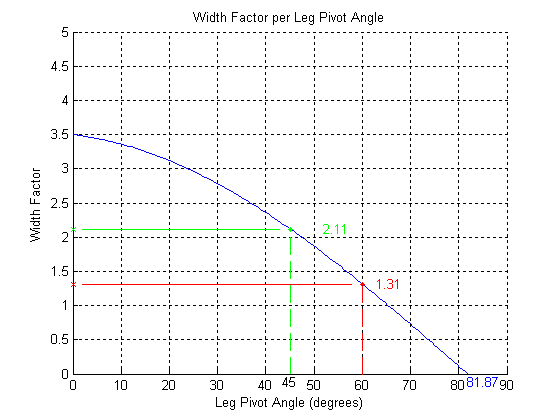Graphs # 1 and # 2 were generated using the following Matlab code: matlab.txt

Figure 21c shows a view of the face of the module core assembly base component with the perimeter of the well as a blue rectangular line. The well perimeter and the surface plane of the face that it intersects with forms the well perimeter edge. The green rectangle represents the leg thickness and rectangular perimeter as a cross section of a leg that can pivot up to 45 degrees, and the red rectangle represents the leg thickness and rectangular perimeter as a cross section of a leg that can pivot up to 60 degrees.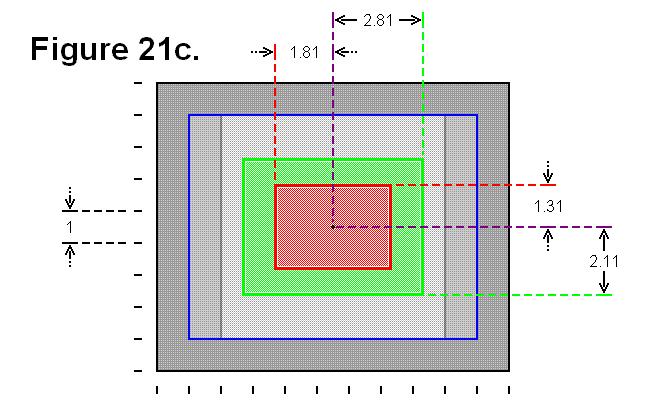In reality, the intermediate universal joint-like component between the module core assembly base component and the first segment of the leg will affect either the shape of the rectangle on the widthwise side of the leg or the shape of the well, because of the cylindrical twisting effect introduced by the universal joint-like component. Also, the universal joint-like component can also affect the lengthwise range, since it pivotally mounts to the module core assembly base component in the region the leg would enter in the well; this might be a limitation that can be minimized or eliminated with an appropriate design for the structure of this universal joint-like component.